### Day 22 - Implicit Differentiation - 02.03.16

 Update Unit 2 TestFriday, 02.12.16Calculus Equations/Theorems Find the derivative of the following.Note: Some of these are problems you have not seen yet.Hint: Try to get y by itself on one side of the equation (if you can).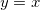xy10none of the above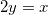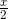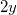20none of the above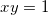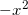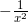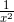none of the above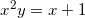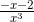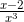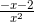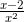none of the above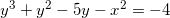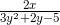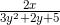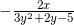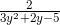impossible to solveReviewPre-calculusSlopeEquation of a LineSecant Line vs. Tangent Line (video)DerivativeHow can the slope of a tangent line be found? Equation of a Tangent Line (video)Tangent Line GraphDerivative (video) (checkpoints)Basic Differentiation Rules (checkpoints)Constant Rule (video) (example)Power Rule (video) (example)Constant Multiple Rule (video) (example)Sum and Difference Rule (video)Derivative of Sine and Cosine FunctionsProduct Rule (video) and Quotient Rule (video) (checkpoints)How can derivatives of the product/quotient of functions be calculated?Chain Rule (video) (checkpoints)How can derivatives of composite functions be found?LessonChallenge 6Find the derivative of the following:Hints will be given as needed.No computers allowed!Implicit Differentiation (video)Checkpoints Exit Ticket Posted on the board at the end of the block.HomeworkStudy! Help Request List Standard(s) APC.2Define and apply the properties of limits of functions.Limits will be evaluated graphically and algebraically.Includes:​limits of a constant​limits of a sum, product, and quotient​one-sided limits​limits at infinity, infinite limits, and non-existent limitsAPC.3Use limits to define continuity and determine where a function is continuous or discontinuous.Includes:​continuity in terms of limitscontinuity at a point and over a closed interval​application of the Intermediate Value Theorem and the Extreme Value Theorem​geometric understanding and interpretation of continuity and discontinuity